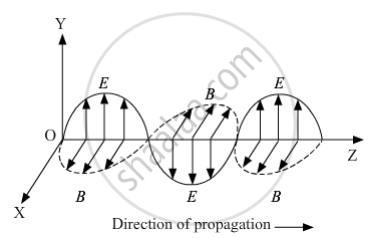# Write Maxwell'S Generalization of Ampere'S Circuital Law. - Physics

Write Maxwell's generalization of Ampere's circuital law. Show that in the process of charging a capacitor, the current produced within the plates of the capacitor is I=varepsilon_0 (dphi_E)/dt,where ΦE is the electric flux produced during charging of the capacitor plates.

#### Solution

A moving charge produces both electric and magnetic fields, and an oscillating charge produces oscillating magnetic and electric fields. These oscillating electric and magnetic fields with respect to space and time produce electromagnetic waves.

The propagation of electromagnetic waves can be shown asOR

Maxwell's generalisation of Ampere's circuital law is given as follows:

oint vec"B".vec"dl"=mu_0(I+I_D)=mu_0(I+varepsilon_0 (dphi)/dt)

Consider that a parallel capacitor C is charging in a circuit.

The magnitude of electric field between the two plates will be E=q/(varepsilon_0 A)and is perpendicular to the surface of the plate.

phi_E=vecE.vecA=EA cos0=q/(varepsilon_0 A)xxA=q/varepsilon_0

=>(dphi_E)/dt=(d(q/varepsilon_0))/dt

=>(dq)/dt=varepsilon_0(dphi_E)/dt ("Here, dq/dt is rate of change of charge with time.")

=>I=varepsilon_0(dphi_E)/dt

Concept: Ampere’s Circuital Law
Is there an error in this question or solution?